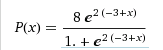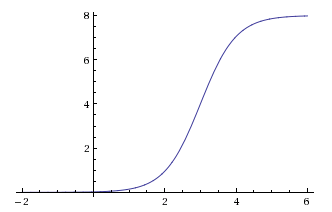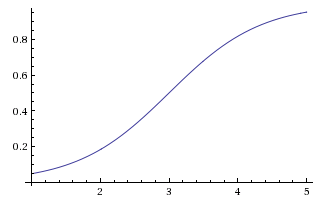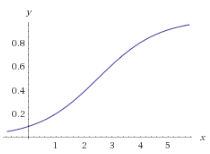avoid Use instead Capitalism Oligopoly Corporate Capitalism Propaganda Persuasion Free Market Quasi Market Society X Cause Y   this is really ambiguous The more X then the more probable is Y X is sufficient cause for Y X is a necessary cause for Y X is a contributory cause for Y independent/ dependent Variables Explanatory/Explained variables endogenous/ exogenous state variables

=============================================

Logistic Function

A general example: If have the phenomena with:

dy/dx=ry(M-y)/M

Then we solve:the solution is:

y(x) = (M e^(c_1 M+r x))/(e^(c_1 M+r x)-1)and we want  y(1)=M/4with 4 categories we like Percentage of M at the center=Pc=Cp(2)=.5MThe solution is:y(x) = (M e^(r x))/(e^(r x)+e^(2 r))=M/(e^(2 r-r x)+1)=In probability cases M=1 and with 4 categories we want p(1)=.25r = 1.09861
y(x)=1/(1+e^(1.09861*(2-x))y(x) = (M e^(r x))/(e^(r x)+3)
y(x) = (M e^(r x))/(e^(r x)+(1/Pc-1))

General from Y(x)= (Me^(r(x-center)))/(e^( r (x-center))+(1/Pc-1))

percentage of Maximum value P(x)= (e^(r(x-center)))/(e^( r (x-center))+(1/Pc-1))

plot P(x)=8 (e^(2(x-3)))/(e^( 2 (x-3))+(1/.5-1)) , -2<x<6Max=8, Centered at 3, and value at the center half of 8 and r is 2

CDF for 4 classes 1 to 4 .2,.3,.3,.2

plot CP(x)=1 (e^(1.5(x-3)))/(e^( 1.5 (x-3))+(1/.5-1)) , x from 1 to 5Flat distribution with 5 ordinal categories: .2,.2,.2,.2,.2

P(x)=1 (e^(r(x-2.5)))/(e^( r (x-2.5))+(1/.5-1))=1-1/(1+e^((-2.5+x)))

1-1/(1+e^((4*log(2)/3+x)))

This is the closest we can approximate a flat distribution Cp(x)=1-1/(1+e^((-2.5+x)))

This has 20% of probability of all less than 1 and

40% probability of all less than 2 andP(x)=O(x)/1+O(x)

O(x|c1)=.2/.8=.25

p(x|c1)=.25/(1+.25)=.2

CO(x|c2)=.5

CO(x|c3)=.75

CO(x|c4)=1

cumulative odds CO(x)=e^(r*2.5+rx)

P(x)=e^(-2.5r+rx)/(1+e^(-2.5r+rx))

r=4*log(2)/3

plot P(x)=1 (e^((4*log(2)/3)(x-2.5)))/(e^( (4*log(2)/3) (x-2.5))+(1/.5-1)) , x from 0 to 7logistic model for CDF 5 ordered categories with constant %: .2,.2,.2,.2,.2

p(x)=1/(1+e^(-(4*log(2)/3)(x-2.5))) x from 0 to 6Therefore if we have a good fit of cumulative probability C(x) by

C(x)=e^(-2.5r+rx)/(1+e^(-2.5r+rx))

or

C(x)=1/(1+e^(-r(x-2.5)))

since

C(x)=O(x)/1+O(x)   Odds of cumulative frequency

cumulative O(x)=e^(r*2.5+rx)

the logit of the probability is the logarithm of the odds.

log(O(x))=r*2.5+rx

logit(C(x))=log(C/(1-C))=log(O(x)=a+rx

this would mean no correlation or association.

for every change of x , C increases with the same %  P
(i+1,j)=p(i,j)+const

=========================================

logistic model for CDF 5 ordered categories with constant rate OF GROWTH FOR %: .05,.1,.2,.4,.8

=============================================

1/(1+e^(-x(2-y)))=.4  ,    1/(1+e^(-x(3-y)))=.6

x~~0.81093, y~~2.5

p(x)=1/(1+e^(-0.81093(x-2.5)))   x from 0 to 61/(1+e^(-x(1-y)))=.2  ,    1/(1+e^(-x(4-y)))=.8

x~~0.924196, y~~2.5

y(x)=1/(1+e^(-0.924196(x-2.5)))Let’s assume we have three ordinal categories.

P(x|c1)=.333

p(x|c2)=.333

p(x|c3)=.333

This means that p(y) is independent of x categories

Cp(y|x=1)=.333

Cp(y|x=2)=.6666

Cp(y|x=3)=1

To center the logistic Cp(y) correctly at 1.5 , the cumulative P at the end of the second class must be .666

1-1/(1+e^(r*(x-1.5)))=.66666

solution is r=1.38623

Cp(y|x)=1-1/(1+e^(1.38623*(x-1.5)))

Co(y|x)=Cp(Y|x)/1-Cp(Y|x)=e^(1.38623 x-2.07935)

Ln(Co(Y|x))=.38623 x-2.07935